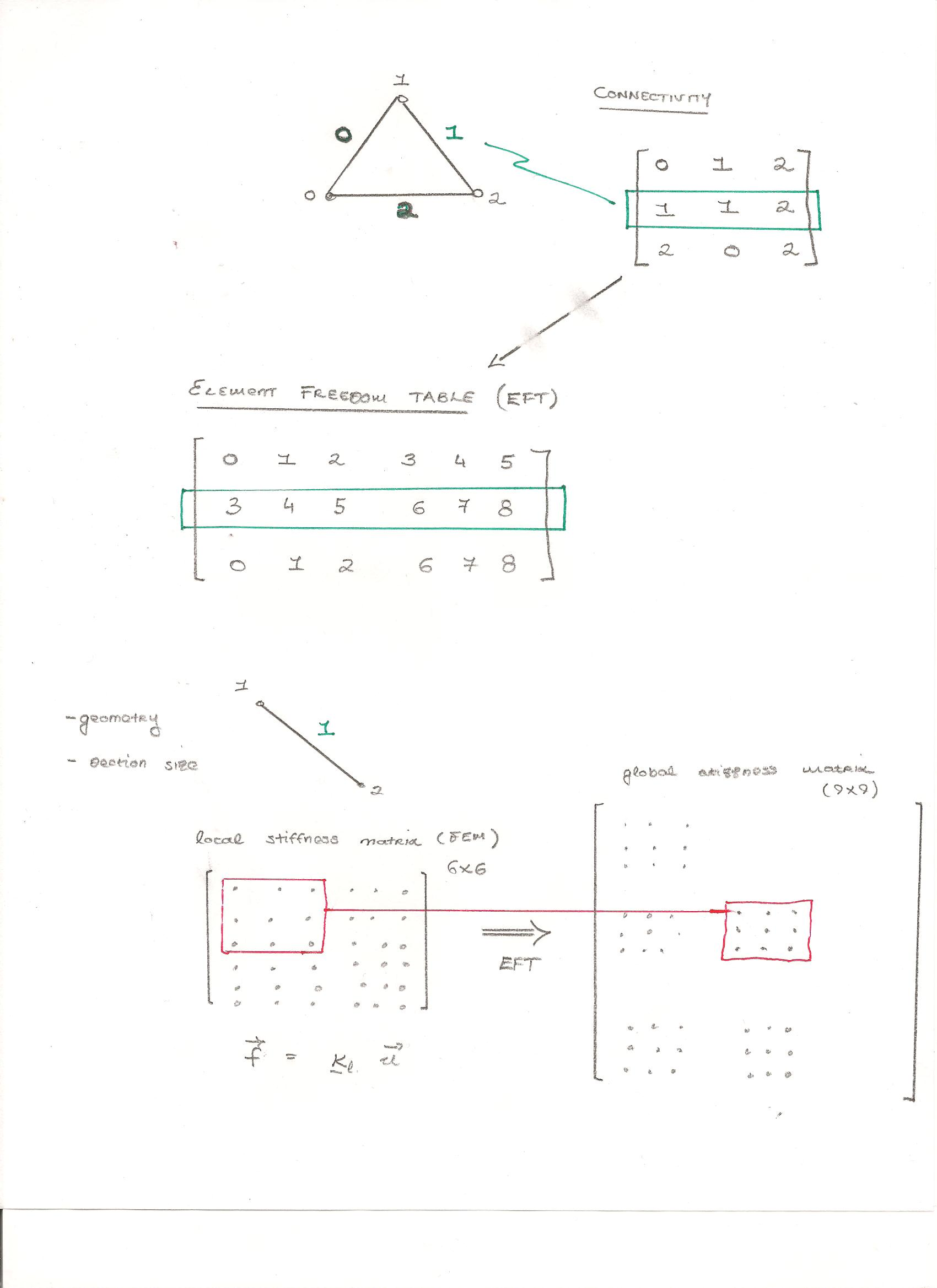Assembly of of overall stiffness matrix from element matrices

When analyzing a range of structures, the way in which the elements connect together will vary. It becomes necessary to provide a method to assemble the local stiffness matrices, built for each element, into the global stiffness matrix for the overall structure. The handwritten notes below show the essence of this approach. A connectivity matrix forms part of the input. This specifies the nodes that each element connects to. The program then constructs an element freedom table which expresses this same information in terms of the degrees of freedom of the problem. The local stiffness matrices, based on Hermite polynomial basis function, are then assembled to form the global stiffness matrix. Fixity (boundary conditions) are then applied, and the matrix is inverted and multiplied by the vector of forces acting on the structure to give displacements of the nodes.